Next: Formalizing Event-Learning in the Up: Illustration: the Event-learning Algorithm Previous: Illustration: the Event-learning Algorithm

## Formal Description of Event-learning

Similarly to most other RL algorithms, the E-learning algorithm [Lorincz et al.(2002)] also uses a value function, the event-value function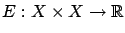. Pairs of states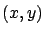and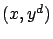are called events and desired events, respectively. For a given initial state, let us denote the desired next state by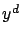. The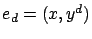state sequence is the desired event, or subtask,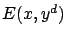is the value of trying to get from actual stateto next state. State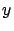reached by the subtask could be different from the desired state. One of the advantages of this formulation is that one may--but does not have to--specify the transition time: Realizing the subtask may take more than one step for the controller, which is working in the background.

This value may be different from the expected discounted total reward of eventually getting fromto. We use the former definition, since we want to use the event-value function for finding an optimal successor state. To this end, the event-selection policy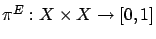is introduced.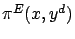gives the probability of selecting desired statein state. However, the system usually cannot be controlled by wishes" (desired new states); decisions have to be expressed in actions. This is done by the action-selection policy (or controller policy)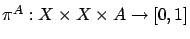, where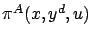gives the probability that the agent selects action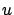to realize the transition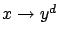.4

An important property of event learning is the following: only the event-selection policy is learned (through the event-value function) and the learning problem of the controller's policy is separated from E-learning. From the viewpoint of E-learning, the controller policy is part of the environment, just like the transition probabilities.

The event-value function corresponding to a given action selection policy can be expressed by the state value function: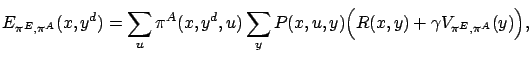and conversely: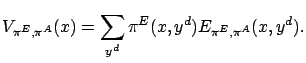From the last two equations the recursive formula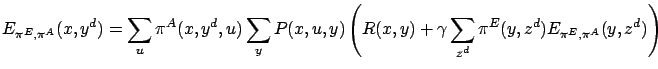(8)

can be derived. Denote by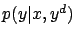the probability that given the initial stateand goal state, the controller and the environment drive the system to statein one step. Clearly,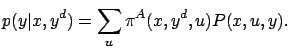(9)

Note that in an on-line process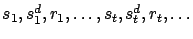(where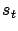,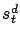and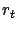denote the actual state, the desired (planned) state and the immediate reward at time step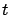), the state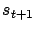is sampled from distribution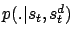. Using Equation 9, Equation 8 can be rewritten in the following form: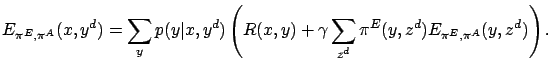Definition 4.1   For a fixed controller policy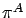, an event-value function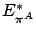is optimal if it satisfies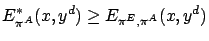for all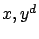and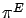.

It can be shown thatsatisfies the following Bellman-type equations: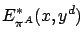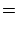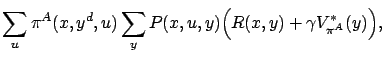where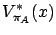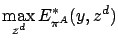Remark 4.2   It is easy to see that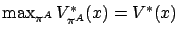. A controller policy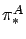is optimal, if it maximizes the l.h.s. of the expression.

An optimal event-value function with respect to an optimal controller policy will be denoted by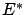.Next: Formalizing Event-Learning in the Up: Illustration: the Event-learning Algorithm Previous: Illustration: the Event-learning Algorithm